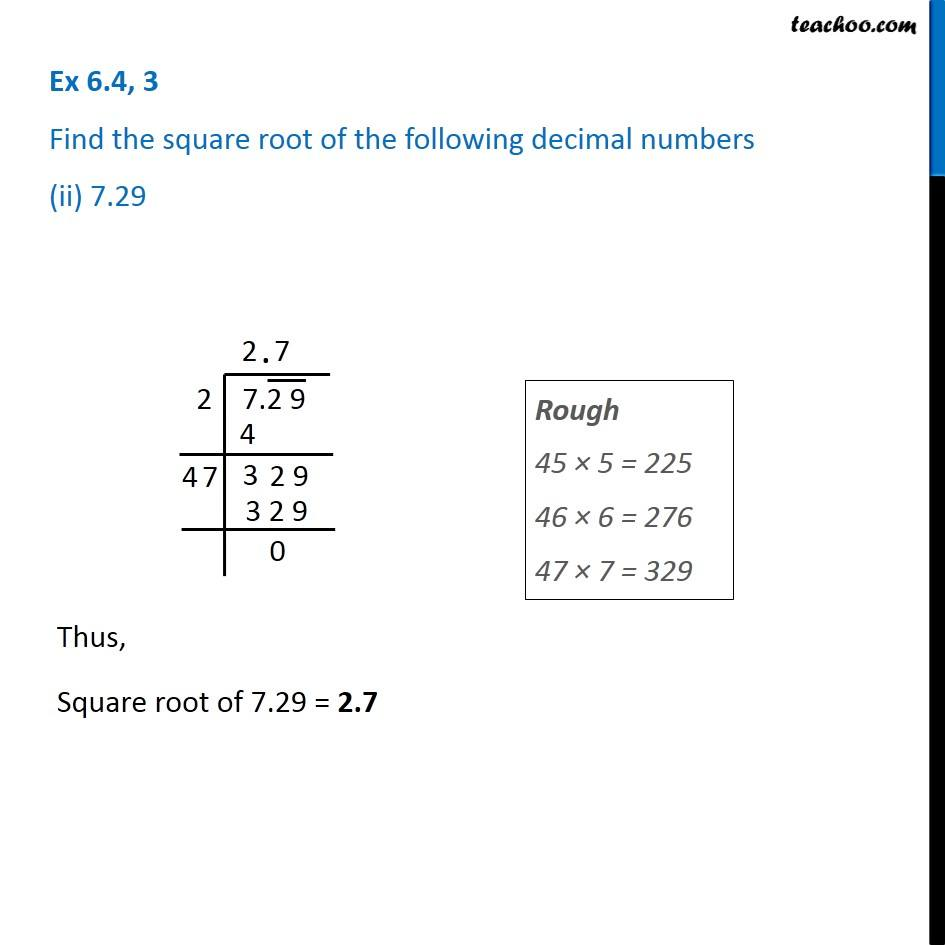1. Chapter 6 Class 8 Squares and Square Roots
2. Serial order wise
3. Ex 6.4

Transcript

Ex 6.4, 3 Find the square root of the following decimal numbers (ii) 7.29 Rough 45 × 5 = 225 46 × 6 = 276 47 × 7 = 329 Thus, Square root of 7.29 = 2.7